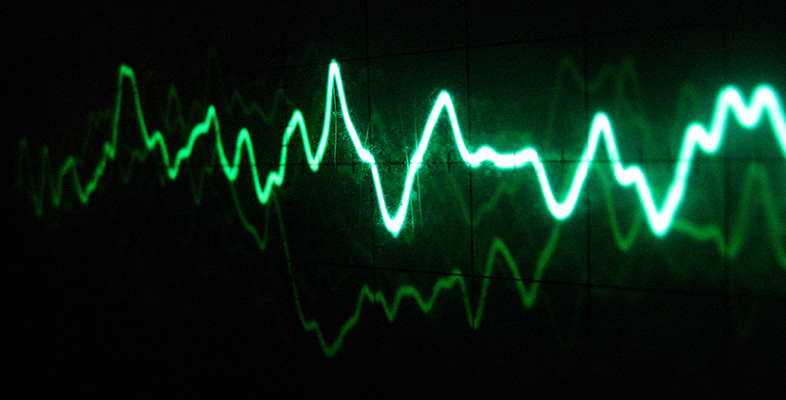Sound for music technology: An introduction

This free course is available to start right now. Review the full course description and key learning outcomes and create an account and enrol if you want a free statement of participation.

Free course

# 6 Amplitude

## 6.1 Defining amplitude

Another important property of a sine wave we need to be able to specify is its amplitude. In essence, the amplitude of a sine wave is its size. Unfortunately there are various ways of defining what is meant by the size of a sine wave, and you are likely to come across many of them in material you look at outside this unit. Before I explain what our definition is, it will help matters if we look at what is meant by the average value of a sine wave.

Figure 16 shows a sinusoidally alternating voltage. The curve is symmetrical around the time axis, which is also the line of zero voltage. The average value of this sine wave over many cycles is therefore zero.Figure 16 Average value of a sine wave is midway between the peaks and troughs

All sine waves are symmetrical around a line running through the middle, midway between the peaks and troughs of the wave. However, it does not follow that all sine waves have an average value of zero. Look at Figure 17. This is an exaggerated graph of a sinusoidal pressure variation in the air.Figure 17 Exaggerated graph of a sinusoidal pressure variation in air: the average value here is not zero

Once again, the average value over many cycles runs midway between the peaks and troughs, but now its value is not zero. The average value is the prevailing atmospheric pressure.

A standard way of defining the amplitude of a sine wave is in terms of its maximum departure from its average value. To see what this means, look at Figure 18.Figure 18 Amplitude for a sine wave of zero average value

The amplitude is the height of the peak relative to the average value. In this case, because the average value is zero, the amplitude is just the peak value of the sine wave, namely 10 volts. However, in Figure 19 the amplitude is not simply the peak value.Figure 19 Amplitude for a sine wave of non-zero average value

Here, because the sine wave does not have an average value of zero, the amplitude is the difference between the peak value and the average value, that is, Pmax − Pa.

In general, the amplitude of a sine wave is the maximum deviation of the sine wave from its average value. In other words, it is the difference between its peak value and its average value. Another way to express this is half the height of the wave from peak to peak.

Sometimes the peak-to-peak height itself is used as a measure of a sine wave's size, because it is easy to read from graphical displays. Such a reading is invariably referred to as the peak-to-peak amplitude, and is twice the amplitude as defined above. It is marked on Figures 18 and 19.

Some authors give a rather different definition of the word ‘amplitude’ from the one given above. For them, the moment-by-moment deviation of a sine wave from its average value is its amplitude. In this usage, the amplitude is constantly changing, and can be specified only at a particular moment. In Figures 18 and 19, a marks an amplitude according to this particular usage. Although we shall not be adopting this usage in this unit, you can expect to encounter it if you look at other books on the subject.

Earlier in this unit, in Figures 6 and 7, you saw graphs representing sound waves travelling away from a source. The amplitudes of the sine waves were constant. In reality the amplitude of a sound wave must decrease with distance from the source. Figure 20 shows how amplitude can decay with distance from a source.Figure 20 Decaying amplitude of a sound wave
TA212_1

### Take your learning further

Making the decision to study can be a big step, which is why you'll want a trusted University. The Open University has 50 years’ experience delivering flexible learning and 170,000 students are studying with us right now. Take a look at all Open University courses.

If you are new to university level study, find out more about the types of qualifications we offer, including our entry level Access courses and Certificates.

Not ready for University study then browse over 900 free courses on OpenLearn and sign up to our newsletter to hear about new free courses as they are released.

Every year, thousands of students decide to study with The Open University. With over 120 qualifications, we’ve got the right course for you.

Request an Open University prospectus